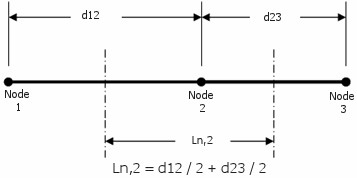# Weld Size Calculations (American Standard)

The software calculates the weld size and weld throat size for all mesh nodes on the intersecting edge of the terminated part based on the following procedure.

1. Establish the local Weld Joint Coordinate System (WJC) at each node.
2. Determine the base material thickness, tb, as the shell thickness of the terminated part.
3. Determine the weld area, Aw, per unit length:
• Single-Sided (SS) welds: Aw = tw, where tw is the weld throat
• Double-Sided (DS) welds: Aw = 2 × tw
4. Determine the weld section modulus, Sw, per unit length:
• DS fillet welds: Sw = tw x tb
• DS groove welds: Sw = (4 / 3) × (tw3 / tb) – 2 × tw2 + tw × tb
• SS fillet or groove welds: Sw = tw2 / 6
5. Calculate the force components and moment based on the local WJC system for each node on the intersecting edge of the terminated part. For DS welds, divide these forces by 2 (the calculations are per unit length of the weld not per unit length of the weld joint).
• Fs = Shear force along the local Us axis.
• Fw = Shear force along the local Uw axis.
• Fj = Normal force along the local Uj axis. Positive Fj values indicate that the top face of the terminated shell is in compression.

• Mw = Nodal bending moment about the local weld axis Uw. Positive Mw values indicate that the top face of the terminated shell is in traction.

6. Calculate the nodal length, Ln, for each node as the sum of ½ the distance to each adjacent node.7. Calculate the stress components per unit length based on the nodal length for each node:
• fs = (Fs / Ln) / Aw
• fw = (Fw / Ln) / Aw
• fj = (Fj / Ln) / Aw + ((Mw / Ln) / Sw)
8. Calculate the resultant stress per unit length on the weld, fweld:
• fweld = sqrt ( fs 2 + fw 2 + fj 2)
9. Solve for the required weld throat, tw, for each node on the intersecting edge of the terminated part by setting fweld = fallowable, where fallowable is the electrode's allowable shear strength.
The allowable shear strength for the electrode's material is calculated as: Ultimate shear strength / factor of safety. Engineering projects governed by specific codes must adhere to the minimums and standards stipulated therein.
10. Calculate the weld size, s, at each node on the intersecting edge of the terminated part:
• SS or DS groove welds: s = tw
• SS or DS 45° fillet welds: s = sqrt(2) × tw